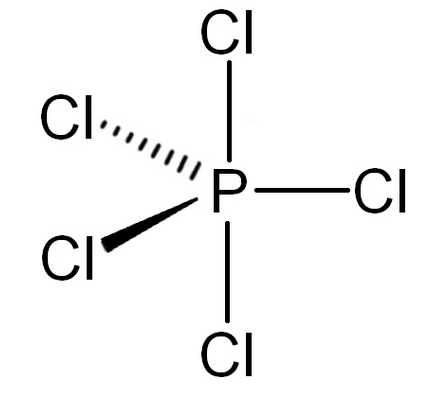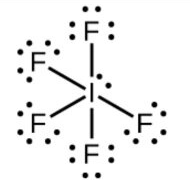Courses
Courses for Kids
Free study material
Free LIVE classes
MoreLIVE
Join Vedantu’s FREE Mastercalss

# $\text{PC}{{\text{l}}_{5}}$ has a shape of trigonal bipyramidal whereas $\text{I}{{\text{F}}_{5}}$ has the shape of a square pyramid. It is due to A. presence of an unshared electron pair on I which is oriented so as to minimise the repulsion while in P in $\text{PC}{{\text{l}}_{5}}$ has no unshared pair.B. octet of P is complete while that of I is incomplete.C. P and I are of different groups.D. F and Cl have different degrees of repulsion.Verified
271.1k+ views
Hint: Phosphorus has five electrons in the outermost shell and it can make five bonds with other molecules whereas iodine has 7 electrons and has a tendency to accept a pair of electrons so it forms mainly a single bond.

-In $\text{PC}{{\text{l}}_{5}}$, the electronic configuration of phosphorus is $\text{1}{{\text{s}}^{2}}\text{ 2}{{\text{s}}^{2}}\text{ 2}{{\text{p}}^{6}}\text{ 3}{{\text{s}}^{2}}\text{ 3}{{\text{p}}^{3}}$ to form five bonds with chlorine electron form s-orbital is excited to the vacant d-orbital due to which phosphorus gains the ability to make 5 bonds.
-Now, it has a total of five unpaired electrons.
-So, the hybridisation of phosphorus is $\text{s}{{\text{p}}^{3}}\text{d}$ and the geometry will be trigonal bipyramidal.
-Because it has 5 bond pairs and 0 lone pairs.-In $\text{I}{{\text{F}}_{5}}$, according to the electronic configuration of iodine two electrons from 5p-orbital are excited to move to the 5d-orbital.
-So, now iodine tends to make 5 bonds and along with it have one lone pair.
-The lone pair will cause the repulsion towards the bond pair and increases the bond angles that’s why it is oriented in such a way that it causes less repulsion.
-So, the hybridisation will be $\text{s}{{\text{p}}^{3}}{{\text{d}}^{2}}$and the geometry will be square pyramidal.
-The structure is:So, the correct answer is “Option A”.

Note: The lone pair- lone pair repulsion causes maximum repulsion than lone pair-bond pair and lone pair-bond pair repulsion is more than the bond pair-bond pair repulsion.
Last updated date: 28th Sep 2023
Total views: 271.1k
Views today: 2.71k